# PSAT Math : How to find the height of a right triangle

## Example Questions

### Example Question #1 : How To Find The Height Of A Right Triangle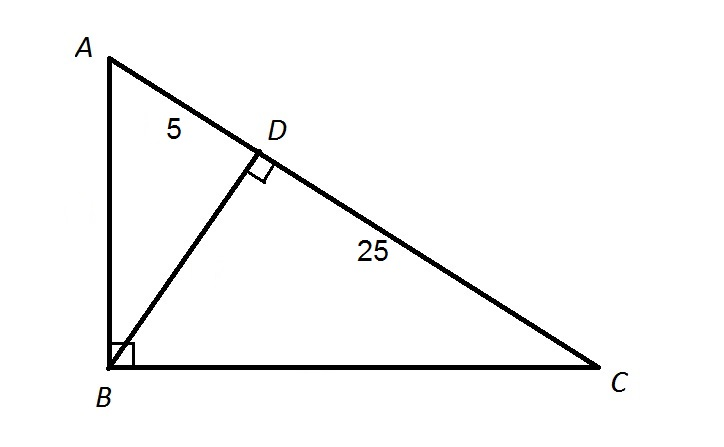Note: Figure NOT drawn to scale.

Refer to the above diagram. Give the ratio of the perimeter of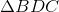to that of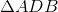.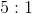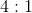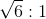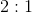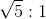Explanation:

The altitude of a right triangle from the vertex of its right triangle to its hypotenuse divides it into two similar triangles.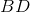, as the length of the altitude corresponding to the hypotenuse, is the geometric mean of the lengths of the parts of the hypotenuse it forms; that is, it is the square root of the product of the two: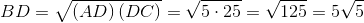.

The ratio of the smaller sides of these similar triangles is

The ratio of the smaller side ofto that ofis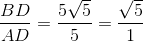or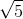: 1,

so this is also the ratio of the perimeter ofto that of.